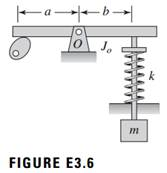### Create an Account

Already have account?

### Forgot Your Password ?

Home / Questions / Derive the governing equation of motion for the rocker arm valve assembly shown in Figure ...

# Derive the governing equation of motion for the rocker arm valve assembly shown in Figure E36 Assume small motions The quantity Jo is the mass moment of inertia about point O of the rocker arm of

Derive the governing equation of motion for the rocker-arm valve assembly shown in Figure E3.6. Assume “small” motions. The quantity Jo is the mass moment of inertia about point O of the rocker arm of length (a + b), k is the stiffness of the linear spring that is fixed at one end, and M is the external moment imposed by the cam on the system. This moment is produced by the contact force generated by the cam at one end of the rocker arm.Jun 25 2020 View more View Less

#### Answer (Solved)Subscribe To Get Solution